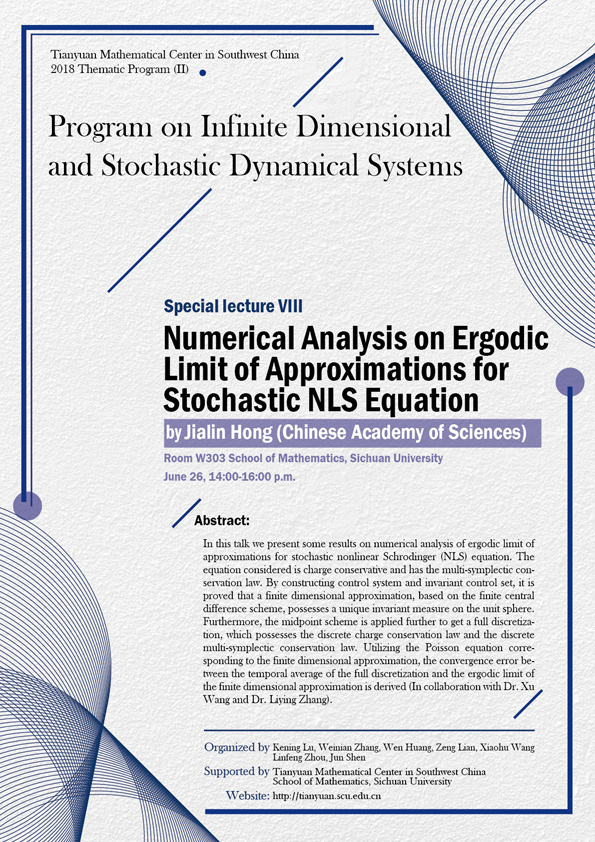2018 Thematic Program (II)

### Numerical Analysis on Ergodic Limit of Approximations for Stochastic NLS Equation

#### W303  School of Mathematics, Sichuan University#### SPEAKER

Jialin Hong (Chinese Academy of Sciences)

#### ABSTRACT

In this talk we present some results on numerical analysis of ergodic limit of approximations for stochastic nonlinear Schrodinger (NLS) equation. The equation considered is charge conservative and has the multi-symplectic conservation law. By constructing control system and invariant control set, it is proved that a finite dimensional approximation, based on the finite central difference scheme, possesses a unique invariant measure on the unit sphere. Furthermore, the midpoint scheme is applied further to get a full discretization, which possesses the discrete charge conservation law and the discrete multi-symplectic conservation law. Utilizing the Poisson equation corresponding to the finite dimensional approximation, the convergence error between the temporal average of the full discretization and the ergodic limit of the finite dimensional approximation is derived (In collaboration with Dr. Xu Wang and Dr. Liying Zhang).

#### ORGANIZERS

Kening Lu (Sichuan University)

Weinian Zhang (Sichuan University)

Wen Huang (Sichuan University)

Zeng Lian (Sichuan University)

Xiaohu Wang (Sichuan University)

Linfeng Zhou (Sichuan University)

Jun Shen (Sichuan University)

#### SUPPORTED BY

Tianyuan Mathematical Center in Southwest China

School of Mathematics, Sichuan University